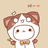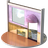首页   注册   登录
V2EX = way to explore
V2EX 是一个关于分享和探索的地方

V2EX  ›  C++

# 拷贝赋值运算符 为啥非要返回个引用

peiqing9003ah · 54 天前 · 1422 次点击

using namespace std;

class A { public : int Aa; int Ab; public: A(int i, int k):Aa(i), Ab(k) {

``````}
A & operator ==(const A& rhs)
{
cout << "这儿有一句傻逼代码" << endl;
return *this;
}
A &  operator >=(const A& rhs)
{
cout << "这儿有另外一句傻逼代码" << endl;
Aa = rhs.Aa;
Ab = rhs.Ab;
return *this;
}
void  operator =(const A& rhs)
{
cout << "这儿有一个拷贝赋值的傻逼代码" << endl;
Aa = rhs.Aa;
Ab = rhs.Ab;
//return *this;
}
``````

}; int main() { std::cout << "Hello World!\n"; A *p1 = new A(1, 3); A *p = new A(2, 3);

``````A b(331,332);
cout << "B" << b.Aa << endl;
cout << "B" << b.Ab << endl;
b = *p;
cout << "B"<<b.Aa << endl;
cout << "B"<<b.Ab << endl;
``````

}

5 回复  |  直到 2019-12-01 00:03:26 +08:001 ifanze   54 天前   ♥ 1 ```A a = xxxx;A b;A c;c = b = a;```2 secondwtq   54 天前 那说明你还需要继续学 C++（或者放弃学 C++）C++ 就是这样，它不会把用户（开发者）当傻逼，你可以做任何事情，它不会阻止你，但是要想玩的转，你最好知道自己在做什么可以这样做 不代表 应该这样做一起努力吧3 c0011   54 天前 这水平就开始看开源代码了？4 coray   54 天前 学 C++不能浮躁...5 yulon   54 天前   ♥ 1 因为赋值运算符的语义就是可以连续赋值的，当然你也可以像标准流的输入输出运算符一样，通过魔改位移运算符来实现，这完全取决于你自己的用途。但是运算符都没学过，就开始玩运算符重载的，也是虎。